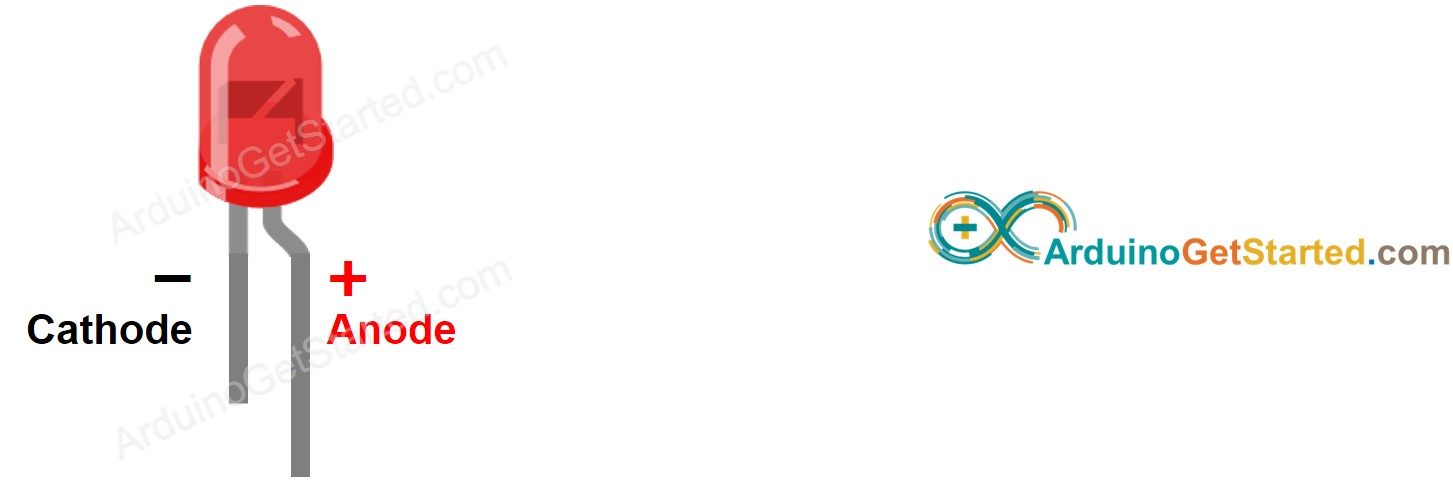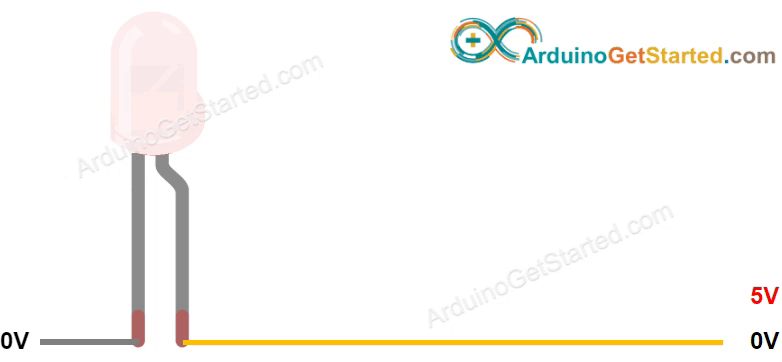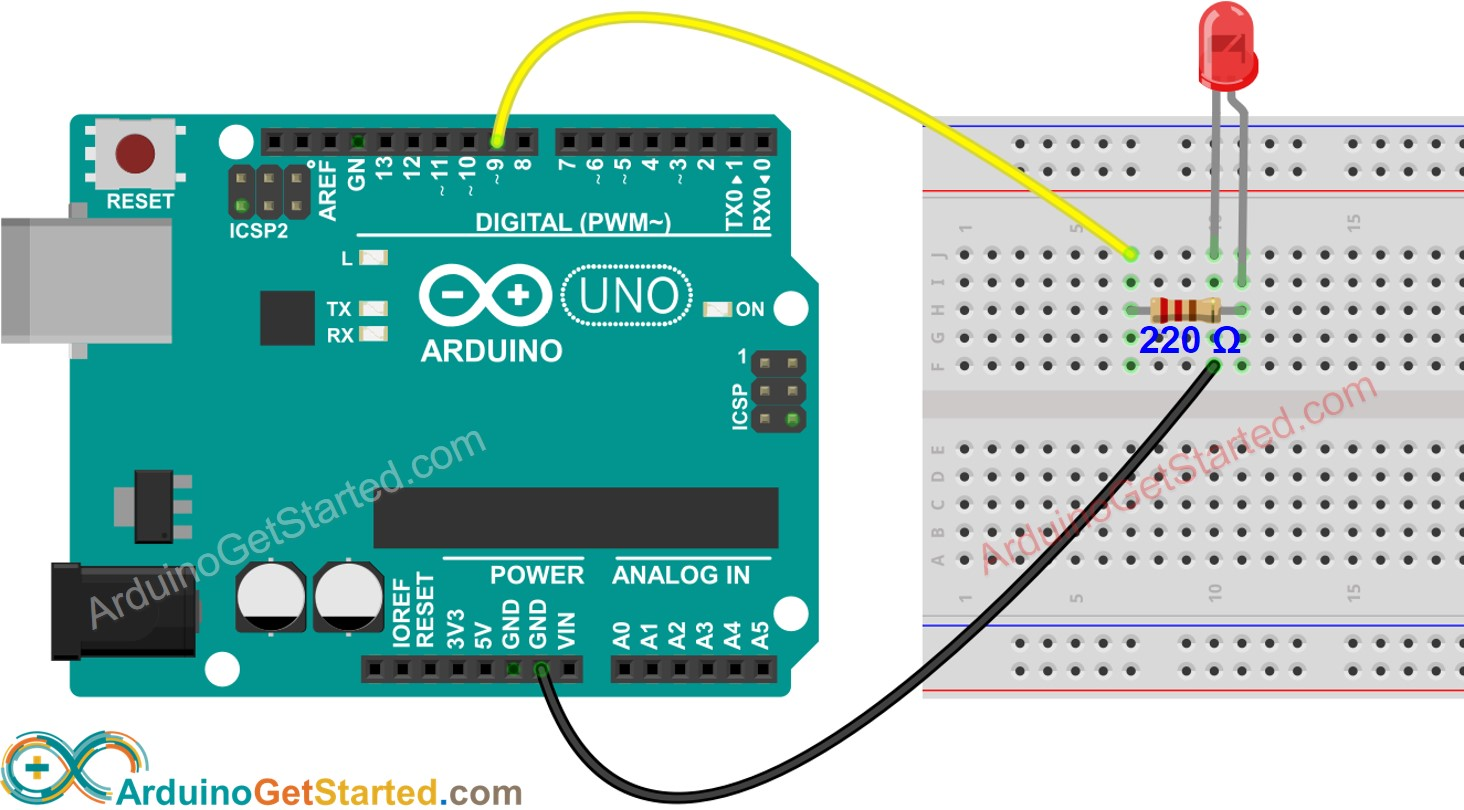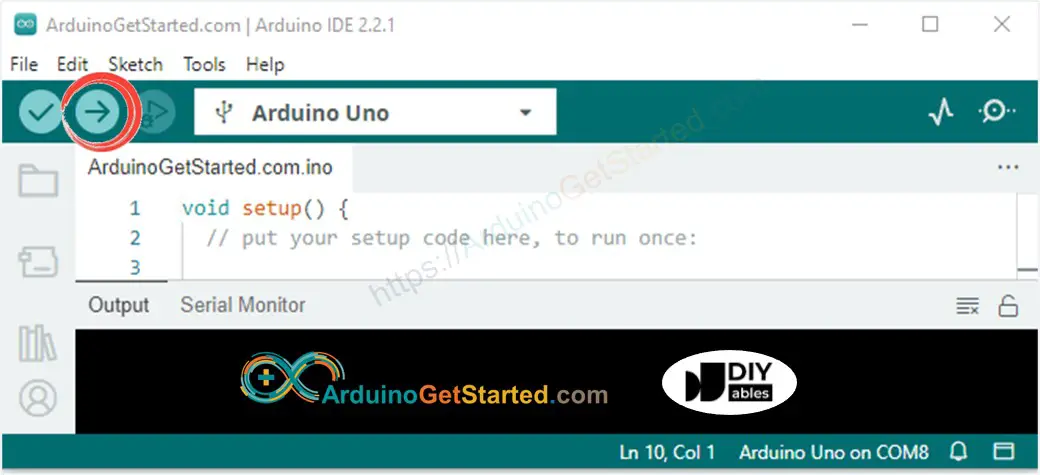# Arduino - LED - Fade

In this tutorial, we are going to learn:

• Arduino fades LED by using delay() function
• Arduino fades LED by using millis() function
• Arduino fades LED by using ezLED library

※ NOTE THAT:

This tutorial provides in-depth knowledge that helps you understand the working principle. To make it easy, you can use Arduino - LED library.

## Hardware Required

Or you can buy the following sensor kit:

Please note: These are Amazon affiliate links. If you buy the components through these links, We will get a commission at no extra cost to you. We appreciate it.

### Pinout

LED includes two pins:

• Cathode(-) pin: needs to be connected to GND (0V)
• Anode(+) pin: is used to control LED's state### How It Works

After connecting the cathode(-) to GND:

• If connecting GND to the anode(+), LED is OFF.
• If connecting VCC to the anode(+), LED is ON.
• If generating a PWM signal to the anode(+), the brightness of LED is changed according to PWM value. PWM value varies from 0 to 255. The bigger PWM value is, the brighter LED is. The smaller PWM value is, the darker LED is.
• If PWM value is 0, it is equivalent to GND, therefore, LED is OFF
• If PWM value is 255, it is equivalent to VCC, therefore, LED is fully ON※ NOTE THAT:

For most of LED, it needs to use a resistor between the anode(+) and VCC. The value of the resistor depends on the specification of LED.

Some of Arduino pins can be programmed to generate PWM signal. We can fade LED by connecting LED's anode(+) pin to an Arduino's pin, LED's cathode(-) to GND, and programming generate PWM on the Arduino's pin.

## Wiring DiagramThis image is created using Fritzing. Click to enlarge image

## How To Program

• Configure an Arduino's pin to the digital output mode by using pinMode() function. For example, pin 9:
pinMode(9, OUTPUT);
• Set brightness of LED by generating the corresponding PWM signal by using analogWrite() function:
analogWrite(9, brightness);

Where the brightness is a value from 0 to 255.

## Arduino Code - Fade Example from Arduino IDE

### Quick Steps

• Connect Arduino to PC via USB cable
• Open Arduino IDE, select the right board and port
• Copy the below code and paste it to the Arduino IDE
/* * Created by ArduinoGetStarted.com * * This example code is in the public domain * * Tutorial page: https://arduinogetstarted.com/tutorials/arduino-led-fade */ #define LED_PIN 9 // the Arduino PWM pin connected to the LED int brightness = 0; // how bright the LED is int fadeAmount = 5; // how many points to fade the LED by // the setup routine runs once when you press reset: void setup() { // declare pin 9 to be an output: pinMode(LED_PIN, OUTPUT); } // the loop routine runs over and over again forever: void loop() { // set the brightness of pin 9: analogWrite(LED_PIN, brightness); // change the brightness for next time through the loop: brightness = brightness + fadeAmount; // reverse the direction of the fading at the ends of the fade: if (brightness <= 0 || brightness >= 255) { fadeAmount = -fadeAmount; } // wait for 30 milliseconds to see the dimming effect delay(30); }
• Click Upload button on Arduino IDE to upload code to Arduino• See the brightness of LED

### Code Explanation

Read the line-by-line explanation in comment lines of code!

※ NOTE THAT:

The above example uses the delay() function to fade-in and fade-out. The delay() function makes the LED fade unsmoothly and blocks other code. In the next parts, we will learn how to fade-in and fade-out smoothly without blocking other code by using millis() function

## Video Tutorial

We are considering to make the video tutorials. If you think the video tutorials are essential, please subscribe to our YouTube channel to give us motivation for making the videos.

## Challenge Yourself

Change the brightness of LED using potentiometer . Hint: Refer to Arduino - Potentiometer.